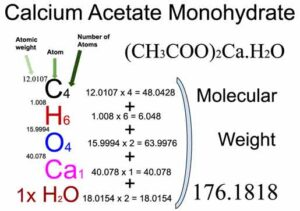# ch3coo2ca

The molecular weight of Calcium acetate monohydrate [(CH3COO)2Ca.H2O] is 176.1818.

Bạn đang xem: ch3coo2caCalcium acetate [(CH3COO)2Ca] is an organic compound of four elements: Carbon, Hydrogen, Calcium, and Oxygen. The monohydrate size of  Calcium acetate [(CH3COO)2Ca.H2O] also contains 1 water molecule. The molecular weight of Calcium acetate monohydrate is 176.1818 which can be calculated by adding up the total weight (atomic weight multiplied by their number) of all its elements Carbon, Hydrogen, Calcium, Oxygen, and water molecule.

# CALCULATION PROCEDURE: Calcium acetate monohydrate [(CH3COO)2Ca.H2O] Molecular Weight Calculation

Step 1: Find out the chemical formula and determine constituent atoms and their number in a Calcium acetate monohydrate molecule.
You will know different atoms and their number in a Calcium acetate monohydrate molecule from the chemical formula. The chemical formula of Calcium acetate monohydrate is [(CH3COO)2Ca.H2O]. From the chemical formula, you can find that one molecule of Calcium acetate monohydrate has four Carbon (C) atoms, six Hydrogen (H) atoms, four Oxygen (O) atoms, one Calcium (Ca) atom, and one water (H2O) molecule.

Step 2: Find out the atomic weights of each atom (from the periodic table).

Atomic weight of Carbon (C): 12.0107 (Ref: Jlab-ele006)
Atomic weight of
Hydrogen (H): 1.008 (Ref: Lanl-1)
Atomic weight of Oxygen (O): 15.9994 (Ref: Jlab-ele008)
Atomic weight of Calcium (Ca): 40.078 (Ref: Pubchem-20, Ciaaw-calcium)
Molecular weight of Water [H2O]: 18.0154

Step 3: Calculate the total weight of each atom in a Calcium acetate monohydrate molecule by multiplying its atomic weight by its number.

Number of Carbon atoms in Calcium acetate monohydrate: 4
Atomic weight of
Carbon (C): 12.0107
Total weight of Carbon atoms in Calcium acetate monohydrate: 12.0107 x 4 = 48.0428

Number of Hydrogen atoms in Calcium acetate monohydrate: 6
Atomic weight of Hydrogen (H): 1.008
Total weight of Hydrogen atoms in Calcium acetate monohydrate: 1.008 x 6 = 6.048

Number of Oxygen atoms in Calcium acetate monohydrate: 4
Atomic weight of Oxygen (O): 15.9994
Total weight of Oxygen atoms in Calcium acetate monohydrate: 15.9994 x 4 = 63.9976

Number of Calcium atoms in Calcium acetate monohydrate: 1
Atomic weight of Calcium (Ca): 40.078
Total weight of Calcium atoms in Calcium acetate monohydrate: 40.078 x 1 = 40.078

Number of water (H2O) molecules in Calcium acetate monohydrate: 1
Molecular weight of water: 18.0154
Total weight of water in Calcium acetate monohydrate: 18.0154 x 1 = 18.0154

Step 4: Calculate the molecular weight of Calcium acetate monohydrate by adding up the total weight of all atoms.

Molecular weight of Calcium acetate monohydrate: 48.0428 (Carbon) + 6.048 (Hydrogen) + 63.9976 (Oxygen) + 40.078 (Calcium) + 8.0154 (Water)= 176.1818

So the molecular weight of Calcium acetate monohydrate is 176.1818.

Calcium acetate monohydrate [(CH3COO)2Ca.H2O] Molecular Weight Calculation

## REFERENCES:

• Lanl-1: https://periodic.lanl.gov/1.shtml
• Jlab-ele006; https://education.jlab.org/itselemental/ele006.html
• Jlab-ele008: https://education.jlab.org/itselemental/ele008.html
• Ciaaw-calcium: https://www.ciaaw.org/calcium.htm
• Jlab-ele020: https://education.jlab.org/itselemental/ele020.html